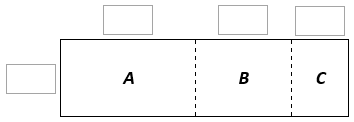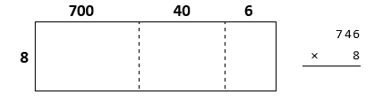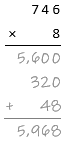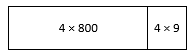# Multi-Digit Multiplication

## Objective

Multiply three-digit numbers by one-digit numbers.

## Common Core Standards

### Core Standards

?

• 4.NBT.B.5 — Multiply a whole number of up to four digits by a one-digit whole number, and multiply two two-digit numbers, using strategies based on place value and the properties of operations. Illustrate and explain the calculation by using equations, rectangular arrays, and/or area models.

?

• 3.NBT.A.3

• 4.NBT.A.1

• 4.NBT.B.4

• 3.OA.B.5

• 3.OA.C.7

## Criteria for Success

?

1. Multiply a three-digit whole number by a one-digit whole number using area models and the partial products algorithm.
2. Estimate products by rounding factors to the largest place value.
3. Solve one-step word problems involving multiplication of three-digit by one-digit numbers (on the Problem Set and Homework) (MP.4).

## Tips for Teachers

?

In Lesson 13, the partial products are listed in decreasing order. This is the way students are likely to write them given the way the area model tends to be set up and because even after their acquisition of fluency, “some students may prefer to multiply from the left because they find it easier to align subsequent products under the biggest product” (NBT Progression, p. 15). Thus, it is included here. But, students will see in Lesson 14 that the partial products can also be written in increasing order. If a student chooses to do so in this lesson, allow them to do so but wait to discuss this with the whole class until Lesson 14.

#### Remote Learning Guidance

If you need to adapt or shorten this lesson for remote learning, we suggest prioritizing Anchor Task 2 (benefits from discussion). Find more guidance on adapting our math curriculum for remote learning here.

#### Fishtank Plus

• Problem Set
• Student Handout Editor
• Vocabulary Package

?

### Problem 1

The area model below can be used to find 7 x 851.To find 7 x 851,

a. Decompose 851 into its base-ten units and write them in the corresponding boxes of the model.

b. Write the area of each region in the model. Then find the area of the entire model. Show your reasoning.

#### References

Open Up Resources Grade 6 Unit 5 Lesson 8 (Teacher Version)Activity 2, "Calculating Products of Decimals"

Modified by The Match Foundation, Inc.

### Problem 2

Ayana used an area model to solve 746 x 8.a. Use the area model to find 746 x 8.

b. Ayana recorded her work in a different way, as shown below.i. In Ayana’s calculation, how are each of the partial products obtained? For instance, where does the 5,600 come from?

ii. How are the partial products in Ayana’s calculation related to the numbers in the area model?

#### References

Open Up Resources Grade 6 Unit 5 Lesson 7 (Teacher Version)Activity 2, "Connecting Area Diagrams to Calculations with Whole Numbers"

Modified by The Match Foundation, Inc.

### Problem 3

Estimate the product. Then use any method to solve.

a. 940 x 6

b. 7 x 589

## Problem Set & Homework

#### Discussion of Problem Set

• Did you draw an area model for #2b? How may sections did it have? Why?
• Look at #2d. What did you estimate the product to be? Do you still think your product is reasonable despite it being hundreds away from the actual product? Why was the estimate hundreds away from the actual product?
• How did you determine what the values of A, B, C, and D were in #4?
• Did you use any strategies from Lesson 11 to solve any problems on today’s Problem Set? For example, #5d?

?

### Problem 1

Ms. Jones is using the area model below to solve a problem.Which equation is represented by the whole area model?

A.   $4\times890=\square$

B.   $4\times809=\square$

C.   $8\times809=\square$

D.   $8\times890=\square$

### Problem 2

Estimate. Then solve. Show or explain your work.

748 x 7

### Problem 3

Isaac ran a lemonade stand all summer. He sold 571 lemonades. If each lemonade cost \$3, how much money did Isaac make?

### Mastery Response

?